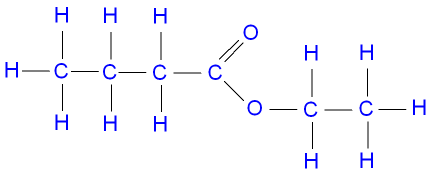# How can I draw the following esters: ethyl butanoate, pentyl propanoate, propyl 3-ethylhexanoate?

Nov 10, 2014

See below.

#### Explanation:

Esters are alkyl alkanoates. They form during the reaction between alcohols and carboxylic acids.

The name of an ester consists of two words.

The first part of the name is the alkyl group of the alcohol.

The second part of the name is the name of the acid minus the ending –ic acid plus the ending –ate.

But we usually write the formula with the acid part first and the alcohol part second.

The acid part of the formula is the original acid minus the carboxylic $\text{H}$ atom: $\text{RCOO-}$.

The alkyl part is $\text{R-}$.

So the formula of the ester is $\text{RCOOR}$.

Ethyl Butanoate

Ethyl is $\text{CH"_3"CH"_2"-}$.

Butanoic acid is $\text{CH"_3"CH"_2"CH"_2"COOH}$. So the butanoate group is $\text{CH"_3"CH"_2"CH"_2"COO-}$

The formula of ethyl butanoate then becomes ${\text{CH"_3"CH"_2"CH"_2"COOCH"_2"CH}}_{3}$

The structural formula looks like this:Pentyl Propanoate

Pentyl is $\text{CH"_3"CH"_2"CH"_2"CH"_2"CH"_2"-}$.

Propanoic acid is $\text{CH"_3"CH"_2"COOH}$. So the propanoate group is $\text{CH"_3"CH"_2"COO-}$

The formula of pentyl propanoate then becomes ${\text{CH"_3"CH"_2"COOCH"_2"CH"_2"CH"_2"CH"_2"CH}}_{3}$

The structural formula looks like this:Propyl 3-Ethylhexanoate

Propyl is $\text{CH"_3"CH"_2"CH"_2"-}$.

Hexanoic acid is $\text{CH"_3"CH"_2"CH"_2"CH"_2"CH"_2"COOH}$. So 3-ethylhexanoic acid is $\text{CH"_3"CH"_2"CH"_2"CH"("CH"_2"CH"_3)"CH"_2"COOH}$

The 3-ethylhexanoate group is $\text{CH"_3"CH"_2"CH"_2"CH(CH"_2"CH"_3)"CH"_2"COO-}$.

The formula of propyl 3-ethylhexanoate then becomes ${\text{CH"_3"CH"_2"CH"_2"CH"("CH"_2"CH"_3)"CH"_2"COOCH"_2"CH"_2"CH}}_{3}$.

The structural formula looks like this: